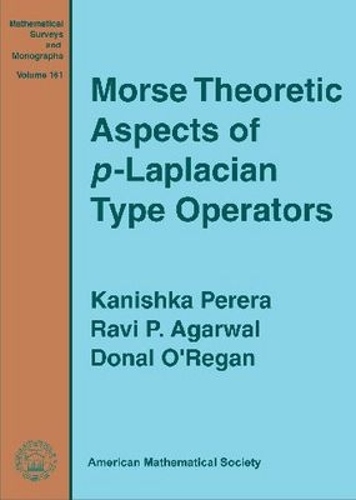•# Morse Theoretic Aspects of \$P\$-Laplacian Type Operators - Mathematical Surveys and Monographs (Hardback)

£76.95
Hardback 202 Pages / Published: 30/05/2010
• We can order this

Usually dispatched within 2 weeks

The purpose of this book is to present a Morse theoretic study of a very general class of homogeneous operators that includes the \$p\$-Laplacian as a special case. The \$p\$-Laplacian operator is a quasilinear differential operator that arises in many applications such as non-Newtonian fluid flows and turbulent filtration in porous media. Infinite dimensional Morse theory has been used extensively to study semilinear problems, but only rarely to study the \$p\$-Laplacian. The standard tools of Morse theory for computing critical groups, such as the Morse lemma, the shifting theorem, and various linking and local linking theorems based on eigenspaces, do not apply to quasilinear problems where the Euler functional is not defined on a Hilbert space or is not \$C^2\$ or where there are no eigenspaces to work with. Moreover, a complete description of the spectrum of a quasilinear operator is generally not available, and the standard sequence of eigenvalues based on the genus is not useful for obtaining nontrivial critical groups or for constructing linking sets and local linkings. However, one of the main points of this book is that the lack of a complete list of eigenvalues is not an insurmountable obstacle to applying critical point theory. Working with a new sequence of eigenvalues that uses the cohomological index, the authors systematically develop alternative tools such as nonlinear linking and local splitting theories in order to effectively apply Morse theory to quasilinear problems. They obtain nontrivial critical groups in nonlinear eigenvalue problems and use the stability and piercing properties of the cohomological index to construct new linking sets and local splittings that are readily applicable here. (SURV/161)

Publisher: American Mathematical Society
ISBN: 9780821849682
Number of pages: 202